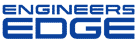# Re: True position calculation

[ Home ][ Forum Archive ] [ Active Engineering Forum ]

Posted ByK. Bramble on April 13, 2002 at 09:14:10:

In Reply to: True position calculation posted byjpeak on April 12, 2002 at 21:27:59:

: Why is true position of a feature calculated as twice the distance (not just the distance)between the dimensioned exact position and the actual position?

What you are talking about is the following equation:

(Position Tolerance) = 2 X Square_root( x^2 + y^2)

In particular you are wondering about the " 2 X " part of the equation.

Postion tolerance for a feature such as a hole is normally expressed as a round zone such as the following (diameter .020), this means that the resultant center axis of the produced hole feature should be manufactured with the limits defined by the round position tolerance (diameter .020). Now, back to the equation, the Square_root( x^2 + y^2) calculates the offset of the measured hole feature center to the theorectical "True postion", this number represents the RADIUS" calculation (center to edge of circle). You then mutilply this number by TWO to get the DIAMETER TOLERANCE ZONE of the actual offset of the measured hole feature.

Hope this helps!

< "> Subject: Re: Re: True position calculation